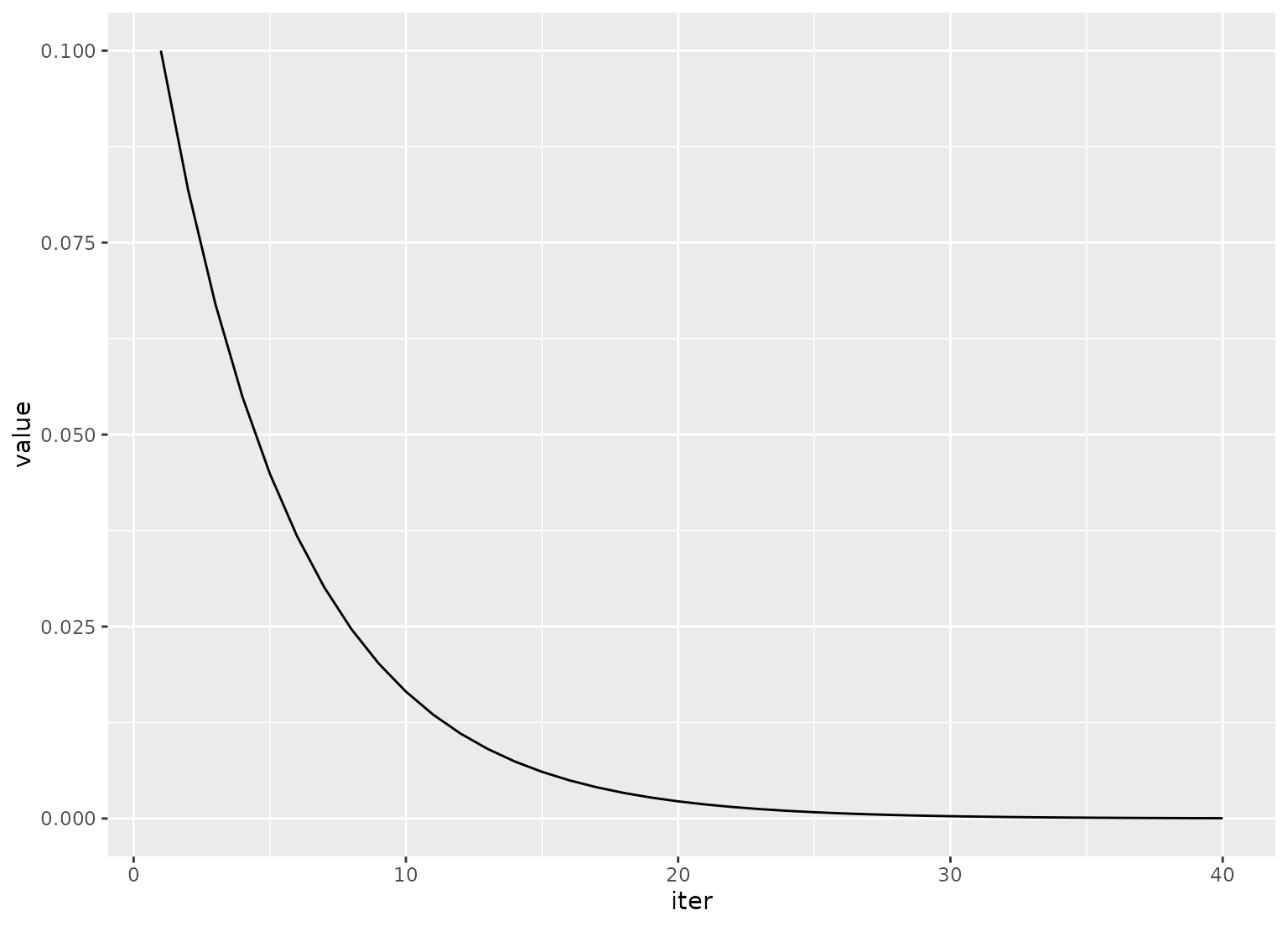expo_decay() can be used to increase or decrease a function exponentially over iterations. This can be used to dynamically set parameters for acquisition functions as iterations of Bayesian optimization proceed.

## Usage

expo_decay(iter, start_val, limit_val, slope = 1/5)

## Arguments

iter

An integer for the current iteration number.

start_val

The number returned for the first iteration.

limit_val

The number that the process converges to over iterations.

slope

A coefficient for the exponent to control the rate of decay. The sign of the slope controls the direction of decay.

## Value

A single numeric value.

## Details

Note that, when used with the acquisition functions in tune(), a wrapper would be required since only the first argument would be evaluated during tuning.

## Examples

library(tibble)
library(purrr)
library(ggplot2)
library(dplyr)
tibble(
iter = 1:40,
value = map_dbl(
1:40,
expo_decay,
start_val = .1,
limit_val = 0,
slope = 1 / 5
)
) %>%
ggplot(aes(x = iter, y = value)) +
geom_path()